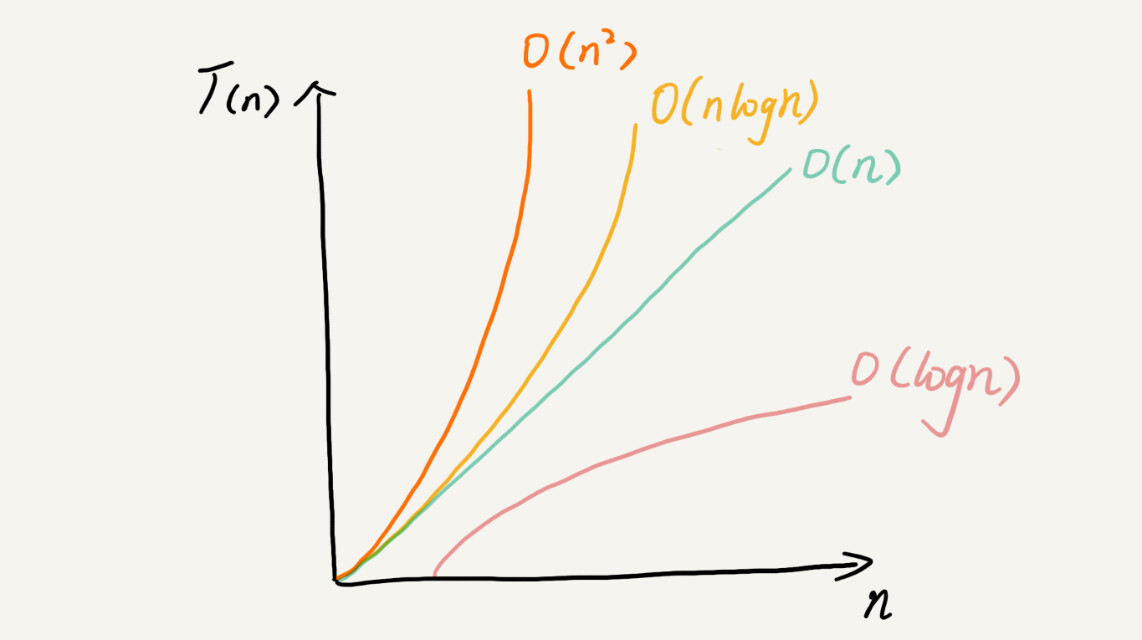##### 时间复杂度

T(n)=O(f(n))



• 看代码中循环次数最多的代码

• 加法法则，总复杂度等于最耗时的那部分代码的复杂度

• 乘法法则 嵌套代码的复杂度等于嵌套内外复杂度的乘积。

 /**
* 最大子序列求和
* O(N^3)
* 算法的思路
* 0 0 0 的最大值
* 0 1 0+1 的最大值
* 0 2 0+1+2 的最大值
* 0 N 0+1+2+。。。N 的最大值
* 1 1 1 的最大值
* 1 1 1+2+。。。N 的最大值
* N N N 的最大值
* 然后对所有的最大值做max
*/
public static int findMaxSubSequenceMax2(int[] a){
int max=0;
for (int i=0;i<a.length;i++){
for (int j=i;j<a.length;j++){
int innerSum=0;
for (int k=i;k<j;k++){
innerSum+=a[k];
}
if (innerSum>max){
max=innerSum;
}
}
}
return max;
}


/**
* 最大子序列求和
* O(N^2)
* a,a+a.....a+a..a[N];取出了本次循环的最大值
* a,a+a.....a..a[N];取出了本次循环的最大值
* 。。。。。
* a[n];取出了本次循环的最大值
* 然后对所有的最大值做max
*/
public static int findMaxSubSequenceMax(int[] a){

int max=0;
for (int i=0;i<a.length;i++){
int innerSum=0;
for (int j=i;j<a.length;j++){
innerSum+=a[j];
if (innerSum>max){
max=innerSum;
}
}
}
return max;
}


/**
* 最大子序列求和
* O(NlogN)
* 采用分治法来处理用到递归计算，递归常用的入参为（数组，上边界，下边界）。作递归要设立基准值
* 处理过斐波那契的都明白需要设定f(0)=1,f(1=1),才有当N>2时候，f(N)=f(N-1)+f(N-2).这里也一样。
* 根据给定的数组，从中间分割为左半部和右半部，最大子序列的和 要么是出现在左半部 要么就是右半部，或者就是中间。
* {[-3],[11,[-5],,,[-7]]},先进行二分就得到下列的小部分数组
*             |
*             V
* {[-3],[11,[-5]}|{,,[-7]]}
*/
public static int findMaxSubSequenceMax3(int[] a,int start,int end){
/**
* 设定基准值,数组中只有一个元素了。那就最大值就是启本身；
*/
if (start==end){
return a[start];
}
/**
* 我们要开始划分小数组了
*/
int mid=(start + end)/2;
int leftMax=findMaxSubSequenceMax3(a,start,mid);
int reghtMax=findMaxSubSequenceMax3(a,mid+1,end);
/**
* 通过上面的我可以知道 左半部分的最大和 右半部分的最大值。但是我并不知道中级部分的数据最大值是什么。
* 那么我就要算出从mid向左走的最大值和向右走的最大值。
*/
/**
* 从mid向左走的最大值
*/
int midLeftMax=Integer.MIN_VALUE,midleftSum=0;
for (int i=mid;i>start-1;i--){
midleftSum+=a[i];
if (midleftSum>midLeftMax){
midLeftMax=midleftSum;
}
}
int midrightMax=Integer.MIN_VALUE,midrightSum=0;
for (int i=mid+1;i<end+1;i++){
midrightSum+=a[i];
if (midrightSum>midrightMax){
midrightMax=midrightSum;
}
}
return Math.max((midLeftMax+midrightMax),Math.max(leftMax,reghtMax));

}


 /**
* 最大子序列求和 联机计算
* O(n)
* 从第一个出发做累加，如果当前值为负数,那么子序列和为0；
* 反之如果这个序列的和大于了max，那么就把当前这个子序列的和赋值给max。
* 循环结束输出max
*/
public static int findMaxSubSequenceMax4(int[] a){
int innerSum=a,max=0;
for (int i=0;i<a.length;i++){

if (innerSum < 0){
innerSum=a[i];
} else{
innerSum+=a[i];
}
if (innerSum>max) {
max = innerSum;
}
}
return max;
}
/**
* 动态规划
* 1. 状态定义
* dp[i] 表示前 i 个元素的最大连续子数组的和
* 2. 状态初始化，数组中第一个元素的最大和就是第一个元素值
* 3. 状态转移
* 转移方程：nums[i] = max(nums[i - 1], 0) + nums[i]
* 更新最大值
*/
public static int findMaxSubSequenceMaxDp(int[] a){
if (a == null || a.length == 0) return 0;
ans = a;
for (int i = 1; i < a.length; i++) {
a[i] = Math.max(a[i - 1], 0) + a[i];
ans = Math.max(ans, a[i]);
}
return ans;
}